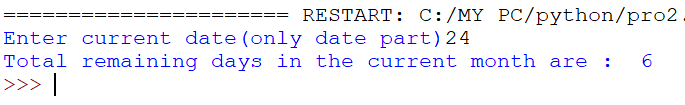# O Level Exam

#### Programming Example

WAP to read todays date (only date Part) from user. Then display how many days are left in the current month.

`Solution`

```import datetime
td=0
day=int(input("Enter current date(only date part)"))
now=datetime.datetime.now()
if now.month==2:
td=28
elif now.month in(1,3,4,7,8,10,12):
td=31
else:
td=30
print("Total remaining days in the current month are : ",td-day)```
`Output`